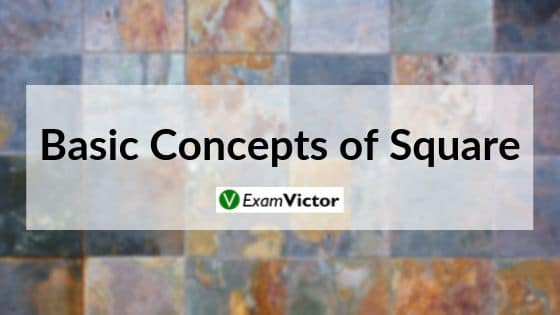# Basics of SquaresLast Updated: Oct 19, 2020

### Some Properties of  Square Numbers

1. If a number has 0 in the unit’s place, then its square ends in 0.
If a number has 1 or 9 in the unit’s place, then its square ends in 1.
2. The square, if, ends in 4, then the number has 2 or 8 in the unit’s place.
3. The number will have 3 or 7 in the unit’s place if the square ends in 9.
4. The number will have 4 or 6 in the unit’s place if the square ends in 6.
5. The square will end in 5 if the number has 5 in the unit’s place.
6. A number ending with one zero will results in 2 zeros in its square. Those ending with two zeros will square itself and give four zeros and so. This means that a perfect square will always end in even number of zeros.
7. The square of a number n is equal to the sum of first n odd natural numbers.
8. The unit’s digit of the square of a natural number is the unit’s digit of the square of the digit at unit’s place of the given natural number.
9. The Square of a natural number other than one is either a multiple of 4 or exceeds a multiple of 4 by 1.

Understanding the basics of square is necessary to solve questions that are asked in various competitive exams in the Quantitative Aptitude section - applicable to CAT, XAT, MAT, SNAP, IIFT, CLAT, AILET, DU LLB, any other entrance exam as well.